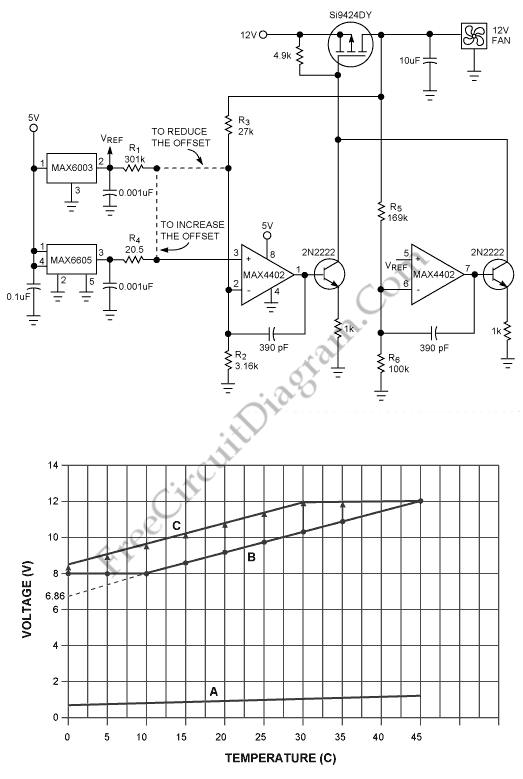# Analog Fan Speed ControlThe large issue today that caused by more electronic equipment enters the home and the office is noise of fan. to reduce fan noise, a variable speed fan is used because it permits quieter and slower, fan speed when temperature conditions allow. To build a variable speed fan we can use digital control or high-speed/low-speed switches. Digitally controlled fans perform well, but those circuits are the system must include a serial bus and more costly. High-speed/low-speed switches are inexpensive, but the sound of sudden speed changes can be annoying. We can use this circuit to solve that problems, it is self-contained analog circuit for fan-speed control and a low cost. Here is the circuit :Curve “A” on Figure 2 represents the output of a MAX6605 analog temperature sensor vs. temperature in °C:

Vsensor = 0.0119V/°C × Temp + 0.744V.

Curve “B” relates the fan voltage to temperature and combines a minimum “floor” voltage of 8.0V with a sloping line:

Vfan = 0.114V/°C × Temp + 6.86V.

We must subtract a voltage offset from the temp sensor output and then multiply the result by a constant to transform line “A” into line “B”. To determine the resistor values we can use this equation:

For the condition R2<<R1,

R1 is any reasonable value,
R2 = R1(AvVtemp0 – Vy-intB)/[(Av-1)(Vref – Vtemp0 +
Vy-intB/Av)], and
R3 = R2(Av-1),

Av = 0.114/0.0119 = 9.58 is the ratio of the desired slope in

V/°C to that of the temperature sensor,

Vtemp0 = 0.744V is the temp-sensor voltage at 0°C,

Vy-intB = 6.86V is the y-intercept indicated by the desired

(extrapolated) temperature curve, and

Vref = 3.0V is the reference voltage.
To calculate the floor voltage we can use this equation:
R5 = R6(Vfloor – Vref)/(Vref), where R6 equals any reasonable value, and

Vfloor = 8V is the desired minimum output voltage.
This equation is used for a desired temperature curve “C” :

Vfan = (0.114V/°C)(Temp + 8.5V),

The required offset gain (8.5V/0.744V = 11.42) is greater than line “B” and the gain (slope) of Av = 9.58 is the same as for line “B”. This equation can be used for such cases:

R4 = R1(Vy-intC/Av – Vtemp0)/(Vref – Vy-intC/Av)
where Vy-intC = 8.5V is the intersection of the desired temperature curve with the y axis. [Source: maxim-ic.com]